# Chapter 10. Functions

In this chapter, the topic of functions will be introduced, together with cases in which they might be useful, as well as the primary concepts when working with functions. It will be demonstrated why it is a good practice to use functions, how to define them and how to call them. The concept of a parameter and a returned value of a function, as well as how we can further use the returned value from the function, will be introduced. At the end of the chapter, we'll look at what the best practices are when dealing with functions.

## What is a Function?

So far, we learned that dividing our code into smaller units (functions), each of which is responsible for a specific functionality is more practical in general. Each unit is concerned with distinct functionality, in which case not only is it easier to process the problem we are facing, but also improves the debugging and readability of the code.

When we think about writing code, the function in practice can be considered as an encapsulated set of instructions that implement the required functionality. This set of instructions (i.e., the function) has its structure, separated from the rest of the code. Throughout the code, we can always call the function when we need its functionality, simply by typing its name and giving appropriate arguments for the parameters if there are any.

A function can be called as many times as we need to solve our problem. This prevents us from typing one functionality several times, which in turn reduces the risk of error if the function is changed afterward.

We will look at two types of functions – "basic functions" (without parameters) and "complex" functions (with parameters).In Object-Oriented Programming (which is out of the scope of this book) the functions are part of higher-level objects called classes, where functions are called methods. In other programming languages, the functions are called procedures.

### Basic Functions

The basic functions are responsible for the execution of an action which contributes to the solving of a particular problem. Such an action can be printing a string to the console, performing a conditional statement check, going through a loop, etc.

Now, let’s look at the following example of a basic function: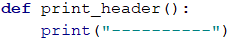The purpose of this function is to print a headline, which, in this case, is a string of dashes. Because of this, it is called 'print_header'. The parentheses always follow the name of the function, irrespective of its name. The names of our functions must describe the purpose of the function. Later in this chapter, we will learn more in-depth about the naming conventions regarding functions.

The function declaration is always followed by a colon, which is followed by the set of instructions (an algorithm), used for solving a particular problem, described by the name of the function. This part of the code is also referred to as the body of the function which contains the machine code (the instructions). It is positioned on a new line after the function’s declaration. It is written with an indentation compared to the function-defining line. The body of the function is always indented four spaces or a single Tab from the level at which the function declaration begins. This separates the body of the function as a single block of instructions.

If the given snippet of code is present in a program that is to be executed, the function will not affect the program at this stage, because the function is declared, but not called yet.

### Why Should We Use Functions?

So far, we found that the functions help with the logical differentiation of a longer code to less complicated, easier-to-digest pieces. Working with functions gives our code advantages, such as better structure and greater cohesion.

The usage of functions prevents us from code repetition. Repeating code is bad practice as it heavily impedes the code’s maintenance and leads to errors. If a single code functionality is present in our program several times throughout the code, and we need to change something to this functionality afterward, we need to correct the same piece of code as many times as we have it in the program. The likelihood of missing one spot in the redaction of the code, in this case, is high, which results in unpredictable behavior. This is the reason why it is a good practice if we do use a fragment of a code more than once, to define it as a separate function.

The functions allow us to use a piece of code several times. Working on more and more problems, you will notice that with the use of already existing functions you will save a lot of time and effort.

### Declaration of Functions

A function declaration can be considered as a registration of a given functionality within a program so that this functionality can be recognized and later used in the rest of the program where necessary. In the next example, we will explore the element of which a function is composed: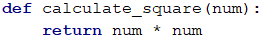• def. The keyword def in Python expresses the intention to declare a new function.
• Name of the function. The name of the function is defined by the developer and follows after the keyword def. It is important to describe the functionality which is bound to be executed by the function’s instructions listed in its body. In our example, the name of the function is calculate_square which hints to us that the given problem aims to calculate the squared value of a number.
• List of parameters. It is introduced in between the parentheses, which we put after the name of the function. Here we list the parameters which we want to include in the function. There might be a single parameter, several parameters, or zero parameters (basic function). If we don’t have a parameter, we leave the parentheses empty. In the function calculate_square there is only one parameter called num.
• Colon. After the closing bracket, we put a colon sign which initiates the beginning of the function’s body.
• Implementation of function (body). The body of the function is the place to put our algorithm (instructions) which solves the problem we deal with. The body, per se, implements the logic of the function. In the particular example, we estimate the squared value of a given number which is num*num. the body is always indented and on a new line after the colon.

Within the definition of a function, it is important to follow this exact structure of the function’s elements.

When declaring a given variable in the body of a function, it is called a local variable for the function. The scope of the variable in which it exists and can be used starts with the line on which we have defined it and spans to the last instruction, part of the function’s body. This space of definition is called the field of scope for the variable.Some programming languages such as C or C++ distinguish between the declaration and the definition of a function. The declaration of a function informs the compiler or the interpreter that a function with its given name exists without imposing implementation. The definition of a function brings in the implementation of the function’s body. Within the Python language, this division into two steps does not exist, which in turn means that each function that is declared must also include some functionality (in the body).

### Invoking a Function

The invoking of the function is regarded as the beginning of the execution of the code which is in the function’s body. This is accomplished as the name of the function is called, followed by the parentheses. If the function expects input arguments for its parameters, they must be inserted in the parentheses in the same order in which the parameters were defined in the parentheses. An example that follows: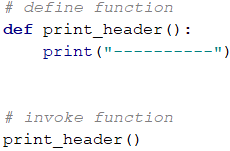The given function can be called plenty of times from any location in the code. It’s important to mention that if a function in Python is defined somewhere inside the code, the program will only recognize it if it is called after its definition within the code of the program.

As the invoking of a function is a command by itself, we can call a function from inside another function: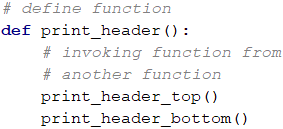There is also a possibility the function to be called in its own body. This is called recursion, for which additional information can be found in Wikipedia and we strongly encourage you to do your research on the internet.

### Problem: Bank Receipt

Write a function that prints an empty receipt. The function should call another three functions: function which prints the headline of the receipt, function which prints the body of the receipt, and function which prints the bottom of the receipt.

Part of receipt Text
Upper part CASH RECEIPT
------------------------------
Middle part Charged to____________________
Lower part ------------------------------
(c) SoftUni

#### Sample Input and Output

Input Outpput
(none) CASH RECEIPT
------------------------------
Charged to____________________
------------------------------
(c) SoftUni

#### Hints and Guidelines

First, we need to create the function which prints the header of the receipt. Its name should be short and sensible which describes the task the function will execute. For example, it can be called print_receipt_header and its body will contain the following code: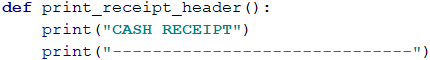Similar to the first function, we will create another two functions which will print out the center part of the receipt (body) and the bottom of the receipt (footer). We will call them print_receipt_body and print_receipt_footer.

Next, we will create another function that wraps the three functions and call them one after another. Finally, we will call the function print_receipt in our program: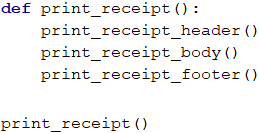#### Testing in The Judge System

The program which contains four functions called inside another function is ready and we can run it and test it locally after which we should send it for a quality check to the Judge platform: https://judge.softuni.org/Contests/Practice/Index/1063#0.

## Functions with Parameters (Complex Functions)

To solve our problem using the function, often in our practice we need additional knowledge which varies with the problem we need to solve. The parameters of a function are supplementing us with this knowledge, hence the behavior of the function depends on these parameters.

### Use of Parameters in a Function

As we just mentioned, the parameters can be zero or as many as needed. When they are declared, commas are used to separate them from each other. The parameters can be of any type (number, string, etc.). The following is an example that demonstrates how exactly the parameters are being used in a body of a function.

This is an example of a definition of a function that uses a set of parameters: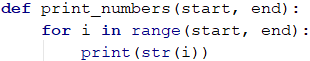In this example, we will be using two parameters called start and end, respectively. After the definition of the function, it can be used in the program – we call the function including arguments for our parameters: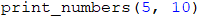In this case, the numerical value of the argument is declared directly in the function (e.g., 5 for start and 10 for the end).

When declaring parameters, they can be of any type (integer, string, etc.) and each parameter has to have a meaningful name. It’s important to notice that when the function is called, the arguments must be put in the same order as the parameters, declared in the definition of the function.

Let’s look at another example of function definition which has a few parameters, each of a different type: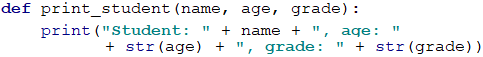### Problem: Sign of Integer Number

Create a function that prints whether the given integer is positive, negative, or zero.

#### Sample Input and Output

Input Output
2 The number 2 is positive.
-5 The number -5 is negative.
0 The number 0 is zero.

#### Hints and Guidelines

The first step is to create a function and give it a descriptive name such as print_sign. This function will have only one parameter – integer number, which sign we want to check:Next, we want to implement the logic which will check whether the input value is positive, negative, or zero. Looking at the examples we can conclude there are three cases to consider – the integer is bigger than zero, the integer is equal to zero and the integer is less than zero, which means we need to check three conditions in the function’s body.

Then we need to read the input value and call our function: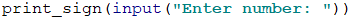#### Testing in The Judge System

Test your solution here: https://judge.softuni.org/Contests/Practice/Index/1063#1.

### Optional Parameters and Default Values

Python allows us to use optional parameters. This in turn allows us to skip initialization of the arguments if default ones are given to the parameters during the function declaration.

The following example will demonstrate the use of default arguments:The given function print_numbers(…) can be called in several ways:On the first line, the parameters use the following arguments – start = 5, end = 10. With the second line the arguments will be - start = 0, end = 15. With the third line we have start = 0, end = 100. The last line will use start = 35, end = 40.

### Problem: Printing Triangle

Create a function that prints triangles as shown in the examples.

#### Sample Input and Output

Input Output Input Output
3 1
1 2
1 2 3
1 2
1
4 1
1 2
1 2 3
1 2 3 4
1 2 3
1 2
1

#### Hints and Guidelines

Before creating a function, that prints numbers on a single line with initial and final values, we must read the input from the console. Next, we choose a meaningful name for our function which shortly describes its purpose. This might be print_line and we can now build it: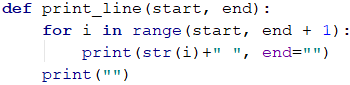Looking at the problems regarding console drawing, we remember that it is a good practice to divide the completion of the figure into several steps. We can simplify the problem by dividing the triangle into three sections – upper, mid, and lower segments.

Our next step will be to print the upper segment of the triangle’s body: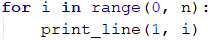After that we need to print the centerline:Finally, we print the bottom segment of the triangle, as this time the step of the for loop is a negative one: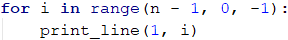#### Testing in The Judge System

Test your solution here: https://judge.softuni.org/Contests/Practice/Index/1063#2.

### Problem: Draw a Filled Square

Draw a filled square to the console with a side length N as it is shown in the examples.

#### Sample Input and Output

Input Output Input Output
4 --------
-\/\/\/-
-\/\/\/-
--------
5 ----------
-\/\/\/\/-
-\/\/\/\/-
-\/\/\/\/-
----------

#### Hints and Guidelines

The first step will be to read the input from the console. Next, we need to create a function that prints the first and the last line as they are identical. Let's not forget that we need to choose a descriptive name and define the length of the side of the square as a parameter: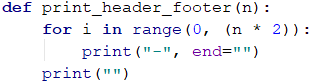The next step is to create a function that draws to the console the lines in the middle. Again, we think of a descriptive enough name, say print_middle_row: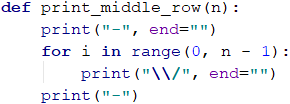Finally, we call the created functions to draw the square: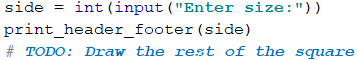#### Testing in The Judge System

Test your solution here: https://judge.softuni.org/Contests/Practice/Index/1063#3.

## Returned Value by a Function

Up until now, we were looking at functions that execute a particular action such as printing some text, value, or a string of characters on the console. Other than such functions, there also can be functions that return a value as a result of their execution – for example, this could the multiplication of two numbers. It will be these functions that will be the topic of our discussion in the next few lines.

### The return Keyword

To return a value that is the result of the function’s execution we use the return keyword. It must be used in the body of the function and indicates to the program to stop the execution of the function and returns value to the caller of the function which is written as an argument after the keyword ‘return’. In the example that follows, there is a function that reads two names from the console, concatenates them, and returns them as a result: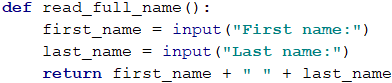The return keyword can be used in functions that do not return anything (the return keyword must be last at the end of the function). The presence of the keyword allows the function to stop its execution without returning a value. In this case, the use of the keyword is helpful only to terminate the execution of the function. It is also possible for the return keyword to be used in more than one place in the function’s body.

In the following example, we have a function that compares two numbers and returns as a result either -1, 0, or 1, depending on whether the first argument is smaller, equal, or bigger than the second argument of the function. The function uses the return keyword on three different instances to return different values depending on the arguments fed to the function.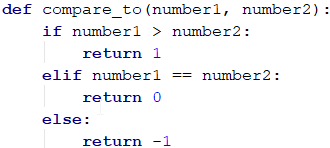It is important to notice that the returned value by the function can be of a different type compared to the argument’s type - string, integer, floating-point number, etc.

#### The Code After return is Unreachable

After the return keyword in a given function, the execution of the function is terminated, and the execution of the program continues where the function was last called. If there are other instructions written after the return keyword, they will not be executed. Some IDEs (including PyCharm) will inform you of the following warning: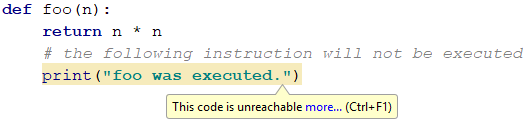In programming, it is meaningless to place two return keywords one after another, as the execution of the first return will not allow the execution of the second return.

### Use Case of a Returned Value from a Function

After a function is executed and the value is returned by its execution, this value can be used in several ways. For once, we can assign this value to a variable: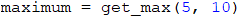Another way to accommodate the returned value is to use it directly in an expression: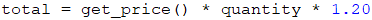The third method of using the returned value of our function is to pass this value to another function via a parameter: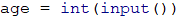### Problem: Calculate Triangle Area

Write a function that calculates the area of a triangle, given the base and height, and returns it as an argument.

Input Output
3
4
6

#### Hints and Guidelines

First, create a function that calculates the surface area based on two input parameters – length of the side a and length of the height h: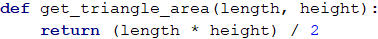The next step will be to read the input values and call the function using them. The result is formatted in an appropriate variable and appears on the screen: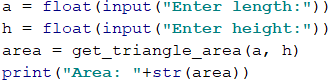#### Testing in The Judge System

Test your solution here: https://judge.softuni.org/Contests/Practice/Index/1063#4.

### Problem: Math Power

First, we need to read the input values from the console. The next step will be to create a function that accepts two arguments as its parameters (a number and its power) and returns, as a result, a float type number.

#### Sample Input and Output

Input Output Input Output
2
8
256 3
4
81

#### Hints and Guidelines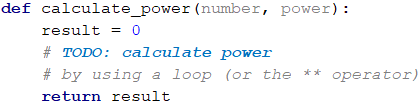After we have done the necessary calculation, we must call the defined function and print the result.

#### Testing in The Judge System

Test your solution here: https://judge.softuni.org/Contests/Practice/Index/1063#5.

### Functions, Returning Several Values

Up to this point, we looked at functions that do not return any value and functions which return only one value. In practice, it is often sensible for a function to return more than one value.

To achieve this in Python, after the return keyword we list the values which are bound to be returned, separated by a comma. The following function accepts as parameters two integers (x and y) and returns two values - the integer and the remainder of their division: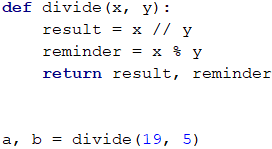Invoking the function works as with functions that do not return a value or return a single value. To use the returned values, we can assign them to variables whose names are separated by commas as demonstrated with a and b. After the execution of this example, a will hold the value 3 while b will hold the value 4.

## Variations of Functions

In many programming languages identically named functions can be used throughout the code using only different parameters which are considered function overloading. Python does not support this functionality, but a similar effect can be achieved with the unconditional parameters introduced earlier.

### Function’s Signature

In programming, the way a function is recognized is by looking at both its fundamental components - name and list of parameters, declared during the definition of the function. These components define its specification, and that's also known as the signature of the function: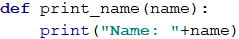In this example, the signature of the function is its name print_name as well as its parameter name.

If in a program we have functions with identical names but different parameters, we are looking at function overloading. This functionality is not implemented in Python.

### Variations of Functions in Python

Different options to call functions in Python can be achieved with the use of default arguments or more precisely with the dynamic allocation of arguments to declared parameters of the function. Let’s look at the example from earlier with a function using a couple of default arguments:As we already noticed, we can call this function using a different combination of arguments that recall closely matched variants of the function:## Problem: Greater of Two Values

The input is two values of the same type. The values can be of int, chr, or str type. Create a function getMax() that returns, as a result, the greater of the two values.

### Sample Input and Output

Input Output Input Output Input Output
int
2
16
16 char
a
z
z string
Ivan
Tod
Tod

### Creating the Functions

We need to create three functions with the same name and different signatures. First, we create a function that will compare integers.

Following the logic of the previous function, we create another one with the same name, but this one will compare characters.

### Testing in the Judge System

Test your solution here: https://judge.softuni.org/Contests/Practice/Index/1063#6.

## Nested Functions (Local Functions)

Let’s consider the following function which calculates the area of a circle: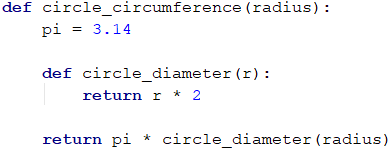### What is a Local Function?

We see that in this piece of code, in the function circle_circumference(…) there is another function called circle_diameter(…). This function is also known as a nested function or a local function. The nested functions can be declared in every other function. They are only visible and can be called in the scope of the function in which they are declared. In the last example, the function circle_diameter(…) can be called only inside the body of the function circle_circumference(…) rather than outside its body.

### Why Using Local Functions?

With practice, we will find that when we write a program, often it appears that we need to use our function only once. Other often faced scenarios are when the function of interest gets too long. We already mentioned that when a function uses too many lines of code, the code gets hard to maintain. In these cases, we can be assisted by the nested functions. They present us with the possibility to declare a function within a function, where a nested function can be used independently. This contributes to the function’s code as it makes it cleaner and improves its readability. This in turn improves the correction time if a bug is spotted later and minimizes the probability of error when changes are made to the code.

### Declaration of a Nested Function

Let’s again have a look at the example from before:In this example, the function cirlce_diameter(…) is nested as it is declared inside the body of the function circle_circumference(…). This means that the function cirlce_diameter(…) can be used only inside the function circle_circumference(…) but not outside of it. If we try to call the function cirlce_diameter(…) outside the function circle_circumference(…) this will cause an error during the execution of the program.

Nested functions have access to all variables which are declared inside the parenting function. In the discussed example pi can be used in the body of the function cirlce_diameter(…). This asset of the nested functions can make them a preferred choice to solve a particular problem. This functionality saves writing time and decreases the amount of code that would otherwise be used to declare parameters and variables, already used in the parenting functions.

## Classic Approaches in The Use of Functions

In this section of the chapter, we'll look at some classic approaches regarding the functions used in a program such as naming conventions, appearance, and structure of the function’s code.

### Naming Conventions

When naming a given function, it is beneficial to use meaningful names. As each function is in charge of some functionality of the problem, it must be taken into account what task it will execute inside the program which makes it a good practice to name the function after the task it executes.

In Python, the convention suggests that the functions must be written in lower cases, as well as that the words used to name the function must be separated by an underscore (_) (if there's more than one word). A good practice is that the function’s name consists of a verb, or a pair of a verb and a noun, respectively.

Some examples of appropriate naming of functions:

• find_student
• load_report
• sine

Some examples of inappropriate naming of functions:

• Method1
• DoSomething
• Handle_Stuff
• SampleMethod
• DIRTYHack

If you can't think of an appropriate name, there is a good possibility that the function is responsible for more than one function or lacks a clearly defined goal. In such cases, it is better to think of how the function can be subdivided into smaller functions.

### Naming Function’s Parameters

In the naming of the parameters of the functions, the same rules apply to the naming of functions. The differences are that it is nice to name a parameter using a noun or a pair of an adjective and a noun, respectively. It should be noted that it is a good practice for the name of the parameter to hint at its type.

Some examples of appropriate naming of parameters:

• first_name
• report
• speed_kmh
• users_list
• font_size_in_pixels
• font

Some examples of inappropriate naming of parameters:

• p
• p1
• p2
• populate
• LastName
• lastName

### More Good Practices in Working With Functions

Let’s again recall how a function should execute only one particular task in the program. If this cannot be achieved, the next thing to do is to look at how to separate the function into a few other functions. As it was said, a name of a function should clearly and precisely state the purpose of the function. Another good practice will be to avoid making the function’s name so long that it can no longer fit on the screen (relatively). If the code in the function gets too large nevertheless, it is recommended to divide the function’s tasks into a few shorter functions within the function as demonstrated:### Structure and Formatting of The Code

In writing functions, caution must be applied to use the correct indentation (one Tab into the function). In Python, incompatible indentation leads to a direct error and does not allow the interpreter to execute the code.

An example of a correctly formatted Python code: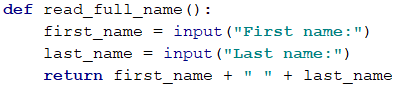An example of an incorrectly formatted Python code (for this reason the last line is underlined in red):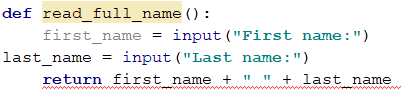Another good rule of thumb is to leave one empty line between the loops and the conditional statements as well as two empty lines after the definition of a function. Also, try to avoid long and complex expressions. Practice shows that these practices improve the readability of the code and save the developer's time.

## What Have We Learned from This Chapter?

In this chapter, we learned the fundamental concepts of working with functions, and:

• Learned that the use of functions comes very useful when larger programs must be fragmented into simpler tasks.
• Explored the structure of a function and how to declare and call it.
• Looked at examples of complex functions and their implementation in a program.
• Discovered what is a signature and returned value as well as the context of the return keyword.
• Get acquainted with the good practices in working with functions – how to name them and their parameters, how to format the code, etc.

## Problems

To consolidate our knowledge about working with functions, a few problems will be solved as an exercise. Each exercise will ask for a function with specific functionality. Then the function must be called, with arguments being passed to the predefined parameters read as inputs from the console. Inputs and outputs beneath each exercise are given as an example.

### Problem: Hello, Name!

Write a function that receives a name as a parameter and prints to the console "Hello, \!".

#### Sample Input and Output

Input Output
Peter Hello, Peter!

#### Hints and Guidelines

Define function print_name(name) and implement its functionality. Then take a string variable (name) as an input argument and call the function, passing the read name.

#### Testing in The Judge System

Test your solution here: https://judge.softuni.org/Contests/Practice/Index/1063#7.

### Problem: Min Method

Create a function called get_min(a, b), which returns the smaller number of the two. Write a program that reads three input values from the console and prints the smallest number. Use the function get_min(…) which is already created.

#### Sample Input and Output

Input Output Input Output
1
2
3
1 -100
-101
-102
-102

#### Hints and Guidelines

Define a function get_min(a, b) and implement its functionality, after which call it as it’s shown. To find the smallest of three numbers, first process one pair, and the result can be combined with the third number such as:

   minimum = get_min(get_min(number1, number2), number3)


#### Testing in The Judge System

Test your solution here: https://judge.softuni.org/Contests/Practice/Index/1063#8.

### Problem: String Repeater

Write a function called repeat_string(str, count), which receives one argument of type string, another argument N of type integer, and returns the string argument N times. The result of the function must be printed to the console.

#### Sample Input and Output

Input Output Input Output
str
2
strstr roki
6
rokirokirokirokirokiroki

#### Hints and Guidelines

Finish the function, as the input argument of type string, must be concatenated to the current output of the loop: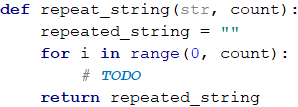#### Testing in The Judge System

Test your solution here: https://judge.softuni.org/Contests/Practice/Index/1063#9.

### Problem: Nth Digit

Write a function find_nth_digit(number, index), which receives a number and index N as parameters and prints the N-th digit from right to left of the number (the first digit from right to left has index 1). The result of the function must be printed on the console.

#### Sample Input and Output

Input Output Input Output Input Output
83746
2
4 93847837
6
8 2435
4
2

#### Hints and Guidelines

To execute the algorithm, we'll use a while loop which is running until the number becomes zero. At each iteration of the while loop, we'll check if the current index of the digit matches with the index passed to the function. If it matches, the digit of the number (number % 10) with this index will be returned from the function. If not, then another digit will be removed from the current number (number = number / 10). Caution must be taken when checking, as the index starts from 1 from right to left. When the current index matches with the given one, the returned value of the function will be the digit from the number with the matched index.

#### Testing in The Judge System

Test your solution here: https://judge.softuni.org/Contests/Practice/Index/1063#10.

### Problem: Integer to Base

Write a function called integer_to_base(number, to_base), which receives as parameters an integer, and a number that indicates the base of the system. The function returns the input number converted to the required numeral system. Next, the result of the function must be printed to the console. The integer will always be of base 10 while the required base will be between 2 and 10.

#### Sample Input and Output

Input Output Input Output Input Output
3
2
11 4
4
10 9
7
12

#### Hints and Guidelines

To solve the problem, a variable will be declared in which the result of the current state of the conversion will be stored as a string. The next steps required for the conversion are as follows:

• Calculate the remainder of the argument’s integer division by the base argument.
• Insert the remainder of the divided argument at the beginning of the predeclared result variable.
• Divide the new argument by the base argument.
• Repeat the algorithm until the current argument becomes 0.

Fill up the missing part of the following function: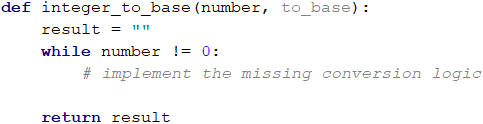#### Testing in The Judge System

Test your solution here: https://judge.softuni.org/Contests/Practice/Index/1063#11.

Write a program that reads an integer N and enters N messages, each of which can be of multiple lines. For each of the messages’ type will be used a different number of parameters. Each message begins with message_type, success, warning, or error:

• When message_type is a success, read operation + message (each on a new line).
• When message_type is a warning, read only the message.
• When message_type is an error, read operation + message + errorCode (each on a new line).

Each read message must be printed to the console, formatted depending on its message_type. After the first line from each output, the next line must be filled with as many equal signs as characters (including spaces) are used on the first line of each printed message. After the last line of each message, an empty line must be included before the beginning of the next message (look at the given output).

In the exercise, you have to use four functions: show_success_message(), show_warning_message(), show_error_message() and read_and_process_message():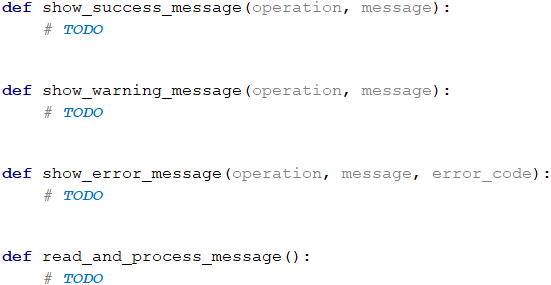#### Sample Input and Output

Input Output
4
error
credit card purchase
500
warning
Email not confirmed
success
user registration
User registered successfully
warning
Customer has no email assigned
Error: Failed to execute credit card purchase.
==============================================
Reason: Invalid customer address.
Error code: 500.

Warning: Email not confirmed.
=============================

Successfully executed user registration.
========================================
User registered successfully.

Warning: Customer has no email assigned.
=========================================

#### Hints and Guidelines

Define and implement the four given functions.

In the function read_and_process_message() the type of the message is read from the console and depending on the type of the message, the remaining elements are also read, which can appear on either one, two, or three consecutive lines, depending on the type of the message. Next, the required function is called to execute the printing of the message.

#### Testing in The Judge System

Test your solution here: https://judge.softuni.org/Contests/Practice/Index/1063#12.

### Problem: * Numbers to Words

Write a function letterize(number), which reads an integer and translates it into words using the following requirements:

• Print each word on the same line (including minus sign if applicable) as shown in the output.
• If the number is bigger than 999, the output must be "too large".
• If the number is smaller than -999, the output must be "too small".
• If the number is negative, minus sign must be printed before the expression.
• If the number does not constitute of three digits, it must not be printed.

#### Sample Input and Output

Input Output Input Output
3
999
-420
1020
nine-hundred and ninety nine
minus four-hundred and twenty
too large
2
15
350
fifteen
three-hundred and fifty
Input Output Input Output
4
311
418
509
-9945
three-hundred and eleven
four-hundred and eighteen
five-hundred and nine
too small
3
500
123
9
five-hundred
one-hundred and twenty three
nine

#### Hints and Guidelines

We can first print the hundreds as a text – (the number / 100) % 10, after that the tens(the number / 10) % 10 and at the end the ones(the number % 10).

The first special case is when the number is exactly rounded to 100 (e.g., 100, 200, 300, etc...). In this case, we print "one-hundred", "two-hundred", "three-hundred" etc.

The second special case is when the number formed by the last two digits of the input number is less than 10 (e.g., 101, 305, 609, etc). In this case, we print "one-hundred and one", "three-hundred and five", "six-hundred and nine", etc.

The third special case is when the number formed by the last two digits of the input number is larger than 10 and smaller than 20 (e.g., 111, 814, 919, etc.). In this case, we print "one-hundred and eleven", "eight-hundred and fourteen", "nine-hundred and nineteen", etc.

#### Testing in The Judge System

Test your solution here: https://judge.softuni.org/Contests/Practice/Index/1063#13.

### Problem: * String Encryption

Write a function encrypt(letter), which encrypts a given letter in the following way:

• It takes the first and the last digit from the ASCII code of the letter and concatenates them into a string, which will represent the result.
• At the beginning of the string, which represents the result, we will insert the symbol which matches the following condition:
• ASCII code of the letter + the last digit of the ASCII code of the letter.
• After that, at the end of the string, which represents the result, you concatenate the character which matches the following condition:
• ASCII code of the letter - the first digit of the ASCII code of the letter.
• The function should return the encrypted string.

Example:

• j → p16i
• ASCII code of j is 106 → First digit - 1, last digit - 6.
• Concatenate the first and the last digit → 16.
• At the beginning of the string, which represents the result, concatenate the symbol, which you get from the sum of the ASCII code + the last digit → 106 + 6 → 112 → p.
• At the end of the string, which represents the result, concatenate the symbol, which you get from subtracting the ASCII code – the first digit → 106 - 1 → 105 → i.

Using the function shown above, write a function that takes a sequence of characters, encrypts them, and prints the result on one line.

The input data will always be valid. The Main function must read the data given by the user – an integer N, followed by a character for each of the following N lines.

Encrypt the symbols and add them to the encrypted string. In the end, as a result, you must print an encrypted string as in the following example:

• S, o, f, t, U, n, i → V83Kp11nh12ez16sZ85Mn10mn15h

#### Sample Input and Output

Input Output
7
S
o
f
t
U
n
i
V83Kp11nh12ez16sZ85Mn10mn15h
Input Output
7
B
i
r
a
H
a
x
H66<n15hv14qh97XJ72Ah97xx10w

#### Hints and Guidelines

Our variable result, in which we will save the value of the result, we will give the initial value "" (empty string). We must run a loop n times so that in each iteration we can add the encrypted symbol to the result string.

To find the first and the last digit of the ASCII code, we will use the same algorithm that we used to solve "N-th number" problem, while to create the string, we will proceed as in "Number to a numeral system"

#### Testing in The Judge System

Test your solution here: https://judge.softuni.org/Contests/Practice/Index/1063#14.